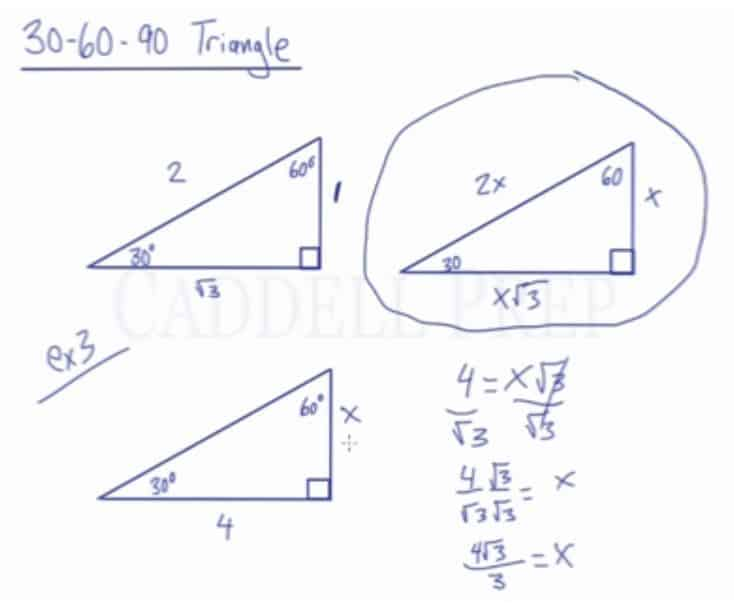In this video, we are going to look at 30-60-90 degree triangles and the ratio that exists between the sides.

30-60-90 represents the angle measurements of a right triangle. This type of triangle is a scalene right triangle. The sides are in the ratio of$1:2:\sqrt3$, with the$1$ across from the 30, the$2$ as the hypotenuse, and the$\sqrt3$ across from 60.

Using variables, it can be written as$x:2x:x\sqrt3$. These relationships can be used to find the other sides of the same special triangle when only given one or two sides.## Video-Lesson Transcript

In this lesson, we’ll review 30-60-90 triangle.

30-60-90 represents the angle measurement of the triangle.

We have one angle at$30^{\circ}$, one angle at$60^{\circ}$, and an angle at$90^{\circ}$.

An example of a 30-60-90 triangle, is a triangle that has a leg measurement of$1$, a hypotenuse of$2$, and another leg is$\sqrt{3}$.

You can plug it in the Pythagorean theorem and see that it works.$1^{2} + \sqrt{3}^{2}$ will end up$2^{2}$.

It’s important that$1$ is across the$30^{\circ}$ angle,$60^{\circ}$ has the$\sqrt{3}$ across from it, and$2$ is the hypotenuse opposite the right angle.

Since it has this proportion, we can have any 30-60-90 triangle being multiple of these sides.

We can double one leg and make it$2$, double the hypotenuse and make it$4$, and$2 \times \sqrt{3}$ on the other leg.

Or simply, one leg can be multiplied by any number so multiply it by$x$ we’ll have$1x$ or simply$x$. The hypotenuse can be multiplied as$2x$. And the other leg will be$x\sqrt{3}$.

This is very important in 30-60-90 triangles.

By knowing this, we can find out any of these sides if we’re given one of the sides.

For example:

One leg across from$30^{\circ}$ is$5$.

Since we’re given one side, we can find the other two sides.

Let’s look at the diagram.$x = 5$

The hypotenuse is$2x$. So, that side is$2x = 2 \times 5 = 10$

The side down here is the side across from the$60^{\circ}$ and in our diagram this side is$x\sqrt{3} = 5 \sqrt{3}$

So, if we’re given any side, we’ll go back to this diagram determine what$x$ is and plug it in for the other sides.

Another example:

We are given the measurement of hypotenuse which is$4$ and we have to find the other sides.

Hypotenuse is across from the$90^{\circ}$. Let’s look at our diagram and see that it’s$2x$.

So, we have$4 = 2x$

Now we need to solve for$x$.$4 = 2x$$\dfrac{4}{2} = \dfrac{2x}{2}$$2 = x$

The side across$30^{\circ}$ angle is$x$ which makes it$2$.

This side is$x = 2$.

The measure of the side across the$60^{\circ}$ angle is$x\sqrt{3}$.

Since we already solved for$x$, that means this side is$2 \sqrt{3}$

To recap, we’re given a hypotenuse of$4$ and was able to figure out that$x = 2$. Then we’re able to figure out the measure of the other two sides by using our diagram.

Let’s have a third example.

In this example we’re given the side across from the$60^{\circ}$ angle is$4$.

So, we need to determine first what is$x$ and we can figure out what are the measures of the other sides.

If we look at the diagram, the side across from$60^{\circ}$ is$x\sqrt{3}$.

This means we have$4 = x \sqrt{3}$

If we figure out what$x$ is then we’ll know the side opposite of$30^{\circ}$ and the hypotenuse.

Let’s solve$4 = x \sqrt{3}$$\dfrac{4}{\sqrt{3}} = \dfrac{x \sqrt{3}}{\sqrt{3}}$$\dfrac{4}{\sqrt{3}} = x$

We’ll never use this for our computation because we shouldn’t have a radical in the denominator.

We have to do something called “rationalizing”.

In order to do that, we have to$\dfrac{4}{\sqrt{3}} \times \dfrac{\sqrt{3}}{\sqrt{3}} = x$$\dfrac{4 \sqrt{3}}{3} = x$

Now that we have$x$, we can find the other sides.

The side across from$30^{\circ}$ is$x = \dfrac{4 \sqrt{3}}{3}$.

The hypotenuse is$2x$.$2 \times \dfrac{4 \sqrt{3}}{3}$$= \dfrac{8 \sqrt{3}}{3}$

Now, we’re able to figure out all of the sides because we’re given the measure of the leg across from$60^{\circ}$ which is$4$.

The diagram can save us a lot of time in solving the lengths of the sides.

Just by using this proportion, any side we’re given just plug it in the side it corresponds with then solve for$x$ and then we can find the measure of the other sides as well.